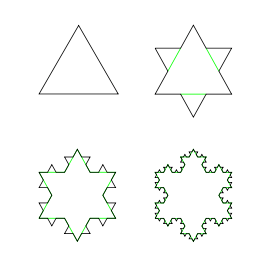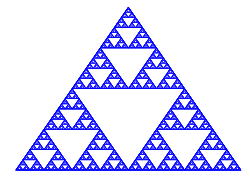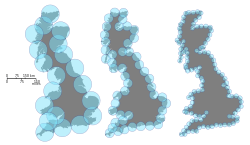# Hausdorff dimensionExample of non-integer dimensions. The first four iterations of the Koch curve, where after each iteration, all original line segments are replaced with four, each a self-similar copy that is 1/3 the length of the original. One formalism of the Hausdorff dimension uses this scale factor (3) and the number of self-similar objects (4) to calculate the dimension, D, after the first iteration to be D = (log N)/(log S) = (log 4)/(log 3) ≈ 1.26. That is, while the Hausdorff dimension of a single point is zero, of a line is 1, of a square is 2, and of a cube is 3, for fractals such as this, the object can have a non-integer dimension.

Hausdorff dimension is a concept in mathematics introduced in 1918 by mathematician Felix Hausdorff, and it serves as a measure of the local size of a set of numbers (i.e., a "space"), taking into account the distance between each of its members (i.e., the "points" in the "space"). Applying its mathematical formalisms provides that the Hausdorff dimension of a single point is zero, of a line is 1, and of a square is 2, of a cube is 3. That is, for sets of points that define a smooth shape or a shape that has a small number of corners—the shapes of traditional geometry and science—the Hausdorff dimension is a counting number (integer) agreeing with a dimension corresponding to its topology. However, formalisms have also been developed that allow calculation of the dimension of other less simple objects, where, based solely on its properties of scaling and self-similarity, one is led to the conclusion that particular objects—including fractals—have non-integer Hausdorff dimensions. Because of the significant technical advances made by Abram Samoilovitch Besicovitch allowing computation of dimensions for highly irregular sets, this dimension is also commonly referred to as the Hausdorff–Besicovitch dimension.

The Hausdorff dimension is, more specifically, a further dimensional number associated with a given set of numbers, where the distances between all members of that set are defined, and where the dimension is drawn from the real numbers, ℝ, to which two elements have been added, +∞ and −∞ (read as positive and negative infinity, respectively). The set that provides the Hausdorff dimension is called the extended real numbers, R, and a set of numbers where distances between all members are defined is termed a metric space, so that foregoing can be succinctly stated, saying the Hausdorff dimension is a non-negative extended real number (R ≥ 0) associated with any metric space.

In mathematical terms, the Hausdorff dimension generalizes the notion of the dimension of a real vector space. That is, the Hausdorff dimension of an n-dimensional inner product space equals n. This underlies the earlier statement that the Hausdorff dimension of a point is zero, of a line is one, etc., and that irregular sets can have noninteger Hausdorff dimensions. For instance, the Koch curve summarized earlier is constructed from an equilateral triangle; in each iteration, its component line segments are divided into 3 segments of unit length, the newly created middle segment is used as the base of a new equilateral triangle that points outward, and this base segment is then deleted to leave a final object from the iteration of unit length of 4. That is, after the first iteration, each original line segment has been replaced with N=4, where each self-similar copy is 1/S = 1/3 as long as the original. Stated another way, we have taken an object with Euclidean dimension, D, and reduced its linear scale by 1/3 in each direction, so that its length increases to N=SD. This equation is easily solved for D, yielding the ratio of logarithms (or natural logarithms) appearing in the figures, and giving—in the Koch and other fractal cases—non-integer dimensions for these objects.

The Hausdorff dimension is a successor to the simpler, but usually equivalent, box-counting or Minkowski–Bouligand dimension.

## Intuition

The intuitive concept of dimension of a geometric object X is the number of independent parameters one needs to pick out a unique point inside. However, any point specified by two parameters can be instead specified by one, because the cardinality of the real plane is equal to the cardinality of the real line (this can be seen by an argument involving interweaving the digits of two numbers to yield a single number encoding the same information.) The example of a space-filling curve shows that one can even take one real number into two both surjectively (so all pairs of numbers are covered) and continuously, so that a one-dimensional object completely fills up a higher-dimensional object.

Every space filling curve hits some points multiple times, and does not have a continuous inverse. It is impossible to map two dimensions onto one in a way that is continuous and continuously invertible. The topological dimension, also called Lebesgue covering dimension, explains why. This dimension is n if, in every covering of X by small open balls, there is at least one point where n + 1 balls overlap. For example, when one covers a line with short open intervals, some points must be covered twice, giving dimension n = 1.

But topological dimension is a very crude measure of the local size of a space (size near a point). A curve that is almost space-filling can still have topological dimension one, even if it fills up most of the area of a region. A fractal has an integer topological dimension, but in terms of the amount of space it takes up, it behaves like a higher-dimensional space.

The Hausdorff dimension measures the local size of a space taking into account the distance between points, the metric. Consider the number N(r) of balls of radius at most r required to cover X completely. When r is very small, N(r) grows polynomially with 1/r. For a sufficiently well-behaved X, the Hausdorff dimension is the unique number d such that N(r) grows as 1/rd as r approaches zero. More precisely, this defines the box-counting dimension, which equals the Hausdorff dimension when the value d is a critical boundary between growth rates that are insufficient to cover the space, and growth rates that are overabundant.

For shapes that are smooth, or shapes with a small number of corners, the shapes of traditional geometry and science, the Hausdorff dimension is an integer agreeing with the topological dimension. But Benoît Mandelbrot observed that fractals, sets with noninteger Hausdorff dimensions, are found everywhere in nature. He observed that the proper idealization of most rough shapes you see around you is not in terms of smooth idealized shapes, but in terms of fractal idealized shapes:

Clouds are not spheres, mountains are not cones, coastlines are not circles, and bark is not smooth, nor does lightning travel in a straight line.

For fractals that occur in nature, the Hausdorff and box-counting dimension coincide. The packing dimension is yet another similar notion which gives the same value for many shapes, but there are well documented exceptions where all these dimensions differ.

## Formal definitions

### Hausdorff content

Let X be a metric space. If SX and d ∈ [0, ∞), the d-dimensional Hausdorff content of S is defined byIn other words,is the infimum of the set of numbers δ ≥ 0 such that there is some (indexed) collection of ballscovering S with ri > 0 for each i  I that satisfies. (Here, we use the standard convention that inf Ø = .)

### Hausdorff dimension

The Hausdorff dimension of X is defined byEquivalently, dimH(X) may be defined as the infimum of the set of d ∈ [0, ∞) such that the d-dimensional Hausdorff measure of X is zero. This is the same as the supremum of the set of d  [0, ∞) such that the d-dimensional Hausdorff measure of X is infinite (except that when this latter set of numbers d is empty the Hausdorff dimension is zero).

## ExamplesDimension of a further fractal example. The Sierpinski triangle, an object with Hausdorff dimension of log(3)/log(2)≈1.58.
• Countable sets have Hausdorff dimension 0.
• The Euclidean spacen has Hausdorff dimension n, and the circle S1 has Hausdorff dimension 1.
• Fractals often are spaces whose Hausdorff dimension strictly exceeds the topological dimension. For example, the Cantor set, a zero-dimensional topological space, is a union of two copies of itself, each copy shrunk by a factor 1/3; hence, it can be shown that its Hausdorff dimension is ln(2)/ln(3)  0.63. The Sierpinski triangle is a union of three copies of itself, each copy shrunk by a factor of 1/2; this yields a Hausdorff dimension of ln(3)/ln(2)  1.58.
• Space-filling curves like the Peano and the Sierpiński curve have the same Hausdorff dimension as the space they fill.
• The trajectory of Brownian motion in dimension 2 and above has Hausdorff dimension 2 almost surely.Estimating the Hausdorff dimension of the coast of Great Britain
• An early paper by Benoit Mandelbrot entitled How Long Is the Coast of Britain? Statistical Self-Similarity and Fractional Dimension and subsequent work by other authors have claimed that the Hausdorff dimension of many coastlines can be estimated. Their results have varied from 1.02 for the coastline of South Africa to 1.25 for the west coast of Great Britain. However, 'fractal dimensions' of coastlines and many other natural phenomena are largely heuristic and cannot be regarded rigorously as a Hausdorff dimension. They are based on scaling properties of coastlines at a large range of scales; however, they do not include all arbitrarily small scales, where measurements would depend on atomic and sub-atomic structures, and are not well defined.

## Properties of Hausdorff dimension

### Hausdorff dimension and inductive dimension

Let X be an arbitrary separable metric space. There is a topological notion of inductive dimension for X which is defined recursively. It is always an integer (or +∞) and is denoted dimind(X).

Theorem. Suppose X is non-empty. ThenMoreover,where Y ranges over metric spaces homeomorphic to X. In other words, X and Y have the same underlying set of points and the metric dY of Y is topologically equivalent to dX.

These results were originally established by Edward Szpilrajn (1907–1976), e.g., see Hurewicz and Wallman, Chapter VII.

### Hausdorff dimension and Minkowski dimension

The Minkowski dimension is similar to, and at least as large as, the Hausdorff dimension, and they are equal in many situations. However, the set of rational points in [0, 1] has Hausdorff dimension zero and Minkowski dimension one. There are also compact sets for which the Minkowski dimension is strictly larger than the Hausdorff dimension.

### Hausdorff dimensions and Frostman measures

If there is a measure μ defined on Borel subsets of a metric space X such that μ(X) > 0 and μ(B(x, r)) ≤ rs holds for some constant s > 0 and for every ball B(x, r) in X, then dimHaus(X) ≥ s. A partial converse is provided by Frostman's lemma.

### Behaviour under unions and products

Ifis a finite or countable union, thenThis can be verified directly from the definition.

If X and Y are non-empty metric spaces, then the Hausdorff dimension of their product satisfiesThis inequality can be strict. It is possible to find two sets of dimension 0 whose product has dimension 1. In the opposite direction, it is known that when X and Y are Borel subsets of Rn, the Hausdorff dimension of X × Y is bounded from above by the Hausdorff dimension of X plus the upper packing dimension of Y. These facts are discussed in Mattila (1995).

### The Hausdorff Dimension Theorem

Theorem. For any giventhere are uncountable fractals with Hausdorff dimensionin n-dimensional Euclidean space## Self-similar sets

Many sets defined by a self-similarity condition have dimensions which can be determined explicitly. Roughly, a set E is self-similar if it is the fixed point of a set-valued transformation ψ, that is ψ(E) = E, although the exact definition is given below.

Theorem. Supposeare contractive mappings on Rn with contraction constant rj < 1. Then there is a unique non-empty compact set A such thatThe theorem follows from Stefan Banach's contractive mapping fixed point theorem applied to the complete metric space of non-empty compact subsets of Rn with the Hausdorff distance.

### The open set condition

To determine the dimension of the self-similar set A (in certain cases), we need a technical condition called the open set condition (OSC) on the sequence of contractions ψi.

There is a relatively compact open set V such thatwhere the sets in union on the left are pairwise disjoint.

The open set condition is a separation condition that ensures the images ψi(V) do not overlap "too much".

Theorem. Suppose the open set condition holds and each ψi is a similitude, that is a composition of an isometry and a dilation around some point. Then the unique fixed point of ψ is a set whose Hausdorff dimension is s where s is the unique solution ofThe contraction coefficient of a similitude is the magnitude of the dilation.

We can use this theorem to compute the Hausdorff dimension of the Sierpinski triangle (or sometimes called Sierpinski gasket). Consider three non-collinear points a1, a2, a3 in the plane R2 and let ψi be the dilation of ratio 1/2 around ai. The unique non-empty fixed point of the corresponding mapping ψ is a Sierpinski gasket and the dimension s is the unique solution ofTaking natural logarithms of both sides of the above equation, we can solve for s, that is: s = ln(3)/ln(2). The Sierpinski gasket is self-similar and satisfies the OSC. In general a set E which is a fixed point of a mappingis self-similar if and only if the intersectionswhere s is the Hausdorff dimension of E and Hs denotes Hausdorff measure. This is clear in the case of the Sierpinski gasket (the intersections are just points), but is also true more generally:

Theorem. Under the same conditions as the previous theorem, the unique fixed point of ψ is self-similar.

## References

1. MacGregor Campbell, 2013, "5.6 Scaling and the Hausdorff Dimension," at Annenberg Learner:MATHematics illuminated, see , accessed 5 March 2015.
2. The distances, taken together, are called a metric on the set.
3. Larry Riddle, 2014, "Classic Iterated Function Systems: Koch Snowflake", Agnes Scott College e-Academy (online), see , accessed 5 March 2015.
4. Keith Clayton, 1996, "Fractals and the Fractal Dimension," Basic Concepts in Nonlinear Dynamics and Chaos (workshop), Society for Chaos Theory in Psychology and the Life Sciences annual meeting, June 28, 1996, Berkeley, California, see , accessed 5 March 2015.
5. Mandelbrot, Benoît (1982). The Fractal Geometry of Nature. Lecture notes in mathematics 1358. W. H. Freeman. ISBN 0-7167-1186-9.
6. Falconer, Kenneth (2003). Fractal Geometry: Mathematical Foundations and Applications (2nd ed.). John Wiley and Sons.
7. Morters, Peres (2010). Brownian Motion. Cambridge University Press.
8. This Wikipedia article also discusses further useful characterizations of the Hausdorff dimension.
9. Marstrand, J. M. (1954). "The dimension of Cartesian product sets". Proc. Cambridge Philos. Soc. 50 (3): 198–202. Bibcode:1954PCPS...50..198M. doi:10.1017/S0305004100029236.
10. Falconer, Kenneth J. (2003). Fractal geometry. Mathematical foundations and applications. John Wiley & Sons, Inc., Hoboken, New Jersey.
11. Mohsen Soltanifar.(2006).On A sequence of cantor Fractals, Rose Hulman Undergraduate Mathematics Journal, Vol 7, No 1, paper 9,
12. Falconer, K. J. (1985). "Theorem 8.3". The Geometry of Fractal Sets. Cambridge, UK: Cambridge University Press. ISBN 0-521-25694-1.
13. Hutchinson, John E. (1981). "Fractals and self similarity". Indiana Univ. Math. J. 30 (5): 713–747. doi:10.1512/iumj.1981.30.30055.